# GSEB Solutions Class 12 Statistics Part 1 Chapter 1 Index Number Ex 1

Gujarat Board Statistics Class 12 GSEB Solutions Part 1 Chapter 1 Index Number Ex 1 Textbook Exercise Questions and Answers.

## Gujarat Board Textbook Solutions Class 12 Statistics Part 1 Chapter 1 Index Number Ex 1

Section A

Answer the following questions by selecting a correct option from the given options:

Question 1.
Which method is useful to compare the long term variations in the values of the variable?
(a) Chain base method
(b) Laspeyre’s method
(c) Fixed base method
(d) Paasche’s method
(c) Fixed base methodQuestion 2.
Which consumption is used in the calculation of Laspeyre’s index number ?
(a) Consumption of base year
(b) Consumption of current year
(c) Consumption of average year
(d) Consumption of any year
(a) Consumption of base year

Question 3.
Which prices are considered in the construction of the cost of living index number ?
(a) Market price
(b) Wholesale price
(c) Average price
(d) Retail price
(d) Retail price

Question 4.
Which expenditure of items is assigned as weights in the method of family budget?
(a) Expenditure of selected year
(b) Average annual expenditure
(c) Expenditure of base year
(d) Expenditure of current year
(c) Expenditure of base year

Question 5.
Which average is considered as the best average in construction of the index number ?
(a) Harmonic mean
(b) Arithmetic mean
(c) Weighted mean
(d) Geometric mean
(d) Geometric meanQuestion 6.
Which index number gives idea of the standard of living of people ?
(a) Index number of industrial production
(b) Quantity index number
(c) Fisher’s index number
(d) Cost of living index number
(d) Cost of living index number

Question 7.
The price of an item increased by 4.5 times in the current year as compared to the base year. What will be the price index number ?
(a) 45
(b) 450
(c) 550
(d) 350
(c) 550

Question 8.
If the purchasing power of money is 0.75 in the year 2016 with respect to the base year 2015, then what will be the price index number for the year 2016?
(a) 750
(b) 175
(c) 133.33
(d) 275
(c) 133.33

Question 9.
If the cost of living index number for the people of a class is 200 for the year 2016 with respect to the year 2015, which of the following statements is true ?
(a) There is an average 200 per cent rise in ‘the current year prices of the items consumed by that class.
(b) There is an average 100 per cent decrease in the current year prices of the items consumed by that class.
(c) Purchasing power of money is. ₹ 0.5.
(d) The current year prices of the items consumed by that class are stable.
(c) Purchasing power of money is. ₹ 0.5.

Question 10.
If Ip = IF, which of the following statements is true ?
(a) Ip = 2IL
(b) IF = $$\frac{\mathrm{I}_{\mathrm{L}}}{2}$$
(c) IF = Ip = IL
(d) 4IF = IL
(c) IF = Ip = ILQuestion 11.
If the average disposable income of families of a class is ₹ 20000 in the year 2013 and if the cost of living index number of that class for the year 2015 with the base year 2013 is 130, what should be the average disposable income of the families of this class in the year 2015?
(a) ₹ 26000
(b) ₹ 20130
(c) ₹ 20000
(d) ₹ 14000
(a) ₹ 26000

Question 12.
What weight is assigned as expenditure to the price relatives $$\frac{p_{1}}{p_{0}}$$ of the items to obtain the formula for Paasche’s index number ?
(a) p0q0
(b) P1q1
(c) P0q1
(d) P1q0
(c) P0q1

Section B

Answer the following questions is one sentence:

Question 1.
What is a price relative ?
A ratio of price of the commodity in the current year with the price in the base year means price relative.

Question 2.
Which method is more suitable to compare the changes in a variable at two different time periods?
A method of ratio is more suitable to compare the changes in a variable at two different time periods.

Question 3.
If the quantity index number of an item for a certain year is 130, interpret it.
The quantity index number of an item for a certain year is 130 means as compared to base year there is 30 % increase in the quantity of an item.

Question 4.
What is a base year ?
When the value of a variable for the current period is compared with the value of the variable with a fixed period, then this fixed period is called a base year.Question 5.
Write the formula for the conversion of chain base index number into fixed base index number.
The formula for the conversion of chain base index number into fixed base index number is as follows :

Fixed base index number = $$\frac{\left(\begin{array}{c} \text { Current year’s chain } \\ \text { base index number } \end{array}\right) \times\left(\begin{array}{c} \text { Previous year’s fixed } \\ \text { base index number } \end{array}\right)}{100}$$

Question 6.
Define the index number.
The percentage change in the value of a variable associated with any item for the current period compared to its value in a fixed period (base period) is called an index number.

Question 7.
Define the cost of living index number.
The number showing the percentage of relative changes in the cost of living of the people of a certain section of the society in the current year as compared to the base year is called the cost of living index number.

Question 8.
What is weight?
The number associated with items in proportion to their importance is called weight.

Question 9.
What is implicit weight?
The weight which is determined as per the number of varieties of different items selected in the construction of index number is called implicit weight.Question 10.
Name the important basic tests of index numbers.
The important basic tests of index number are:

• Time reversal test, and
• Factor reversal test.

Question 11.
What is a chain base index number?
An index number obtained by comparing the current period with the recent past period is called a chain base index number. It’s formula is as follows :
Chain base index number
= $$\frac{\text { Value of the variable in the current period }}{\text { Value of the variable in the preceding period }}$$ × 100

Question 12.
‘The price index number of oil is ₹ 500.’ State whether this statement is true or false and if false, correct and rewrite it.
‘The price index number of oil is ₹ 500.’ This statement is false.
Correct statement: ‘The price index number of oil is 500’.

Question 13.
Which index number is used to find the rate of inflation ? Write the formula to find the rate of inflation.
The wholesale price index number is used to find the rate of inflation. It’s formula is as follows:
Rate of inflation = $$=\frac{\left(\begin{array}{c} \text { Wholesale price } \\ \text { index number of } \\ \text { current year } \end{array}\right)-\left(\begin{array}{c} \text { Wholesale price } \\ \text { index number of } \\ \text { preceding year } \end{array}\right)}{\text { Wholesale price index number of preceding year }}$$ × 100Question 14.
Which index number is used in India to find the rate of dearness allowance ?
The cost of living index number is used in India to find the rate of dearness allowance.

Question 15.
State the main difference between fixed base method and chain base method.
The main difference between fixed base method and chain base method is that ‘in fixed base method the base year remains constant throughout the period of comparison while in chain base method at any given time the preceding year is taken as the base year.

Question 16.
In which method does the base year change each year ?
In chain base method, the base year changes each year.

Question 17.
Which is the appropriate average for the construction of index number ?
The weighted average is the appropriate average for the construction of index number.

Question 18.
How should be the base year in the calculation of index number ?
The base year in the calculation of index number should be standard or normal free from natural calamities and abnormal man-made events.Section C

Answer the following questions as required:

Question 1.
What is a base year ? Which points should be considered while choosing it?
A fixed period with which the value of a variable for the current period is compared, is called base year.
The following points should be considered while choosing it:

• The base year should be standard or normal.
• It should be free from natural calamities like floods, draught, earthquake, abnormal man-made events like war, revolt, riot, strike, agitation, political events, economic disturbances, etc.
• It should not be from a distant past.
• It should be so selected that reveal the realistic picture of the current situation.
• If a single period is not normal then it should be selected as the average of some past periods.

Question 2.
State the characteristics of index number.
The characteristics of index number are as follows :

• Index number is a relative measure expressing percentage changes in the value of a variable quantity.
• It is independent of units of measurement.
• It is a comparative measure.
• It compares the situations prevailing at two different points of time by means of ratio.
• It is an average measure. Hence, it possesses all characteristics of an average.
• It is a weighted average.

Question 3.
What is quantity index number ?
For a group of n mutually related items if an index number is found using relative change in the quantity of each item in that group, then the average of all such index numbers is called the quantity index numbers for that group.

→ Quantity index number is obtained by the following formula:
Quantity index number = $$\frac{\sum\left(\frac{q_{1i}}{q_{0 i}}\right)}{n}$$ × 100
Where, q1i = Current year’s quantity for ith item (i = 1, 2, 3, …………. n)
q0i = Base year’s quantity for ith item (i = 1, 2, 3, ………….. n)
n = Number of itemsQuestion 4.
What is weight in the construction of index numbers ? State the types of weight.
The number of items selected in the construction of an index number, the importance of every item is generally not same. So a number assigned to each item according to its importance is called weight of the item.

→ The weights assigned to the items are of two types:

1. Implicit weight and
2. Explicit weight.

Question 5.
Why is Fisher’s index number called an ideal number?
Ans.
The Fisher’s index number is called an ideal index number due to the following reasons:

• Fisher’s index number considers and quantities of both the years, base year and current year in the construction of the index number.
• It satisfies both the important fundamental tests, time reversal and factor reversal tests, of index number.
• The geometric mean is used to calculate this index number which is the best average for the construction of index number.
• It balances the demerits of Laspeyre’s and Paasche’s index numbers.Question 6.
State the main difference between explicit weight and implicit weight.
The main differences between explicit weight and implicit weight are as follows:

 Explicit weight Implicit weight 1. Explicit weights are determined according to the importance of the items. For example, in food items if wheat is twice important than rice, then explicit weight of wheat is two and that of rice is one. 1. Implicit weight is implied in the selection of items. The weight is considered according to the varieties of the items selected. For example, if in food items, four varieties of wheat is selected then weight of wheat is four. 2. Explicit weight can be expressed directly in numbers. 2. Implicit weight cannot be expressed in numbers. 3. This is the direct method of assigning weight. Hence, it is decided by the two methods: (1) Total expenditure method and (2) Family budget method. 3. This is an indirect method of assigning weight. Hence, there is no other method of determining it.

Question 7.
The cost of living index number increased from 280 to 340 during a certain time period and the wage increased from ₹ 13500 to ₹ 14750. Find the real gain or loss of the worker.
When cost of living index number is 280, then wage = ₹ 13500
∴ Cost of living index number is 340, then wage
= $$\frac{13500 \times 340}{280}$$
= $$\frac{4590000}{280}$$
= ₹ 16392.86
But the wage of a worker becomes ₹ 14750.
So, the loss of the worker = ₹ (16392.86 – 14750) = ₹ 1642.86Question 8.
The cost of living index numbers and average monthly wage from the year 2010 to 2013 are given as follows. Find the real wage for each year.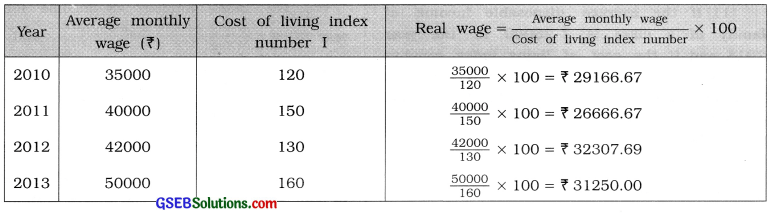Question 9.
The wholesale price index numbers for the year 2014 and 2015 are found to be 177.6 and 181.2 respectively. Find the rate of inflation using index numbers of both the years.
Wholesale price index number for the year 2014 = 177.6 and
Wholesale price index number for the year 2015 = 181.2
Rate of inflation = $$=\frac{\left(\begin{array}{c} \text { Wholesale price index } \\ \text { number for the year } 2015 \end{array}\right)-\left(\begin{array}{c} \text { Wholesale price index } \\ \text { number for the year } 2014 \end{array}\right)}{\text { Wholesale price index number for the year } 2014}$$ × 100
= $$\frac{181.2-177.6}{177.6}$$ × 100
= $$\frac{3.6}{177.6}$$ × 100
= 0.0203 × 100
= 2.03 %Question 10.
The percentage increase in the price relatives of three items are 315, 328 and 390 respectively. If the importance of these items has ratio 5:7 :8, find the general price index number.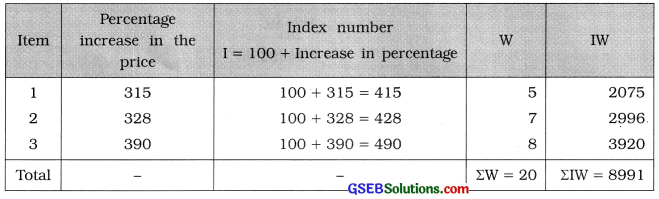General price index number = $$\frac{\Sigma \mathrm{IW}}{\Sigma \mathrm{W}}$$
= $$\frac{8991}{20}$$
= 449.55

Question 11.
If the average disposable income of family of a class is ₹ 25000 in the year 2014 and if the cost of living index number of that class for the year 2016 with the base year 2014 is 120, estimate the average disposable income of the family of that class in the year 2016.
The average disposable income of family of a class = ₹ 25000 in the year 2014.
Base year is 2014. So the cost of living index number for the year 2014 = 100.
Cost of living index number for the year 2016 = 120.
Now, if the index number is 100, then the average disposable income is = ₹ 25000.
∴ If the index number is 120, then the average disposable income = $$\frac{25000 \times 120}{100}$$
= ₹ 30000

Question 12.
The average monthly income of a worker was ₹ 16000 in the year 2015 and it increased to ₹ 20000 in the year 2016. Find the index number of income for the year 2016 in comparison to the year 2015.
The average monthly income of a worker in the year 2015 = ₹ 16000 and in the year 2016 = ₹ 20000.
∴ The index number of income for the year 2016 = $$\frac{\text { Average monthly income in } 2016}{\text { Average monthly income in } 2015}$$ × 100
= $$\frac{20000}{16000}$$ × 100
= 1.25 × 100 = 125Question 13.
If the production of an item increased by JJ times in the year 2016 as compared to the base year, find the index number of production for the year 2016.
Suppose the production of base year = 1
∴ The production in the year 2016
= 1 + (1 × $$\frac{9}{5}$$) = 1 + $$\frac{9}{5}$$ = $$\frac{14}{5}$$
∴ The index number of production for the year 2016 = $$\frac{\frac{14}{5}}{1}$$ × 100
= $$\frac{14}{5}$$ × 100 = 280

Question 14.
If IL = 221.5 and IF = 222, find IP.
IL = 221.5, IF = 222, IP = ?
IF = $$\sqrt{I_{L} \times I_{P}}$$
∴ 222 = $$\sqrt{221.5 \times \mathrm{I}_{\mathrm{P}}}$$
∴ (222)2 = 221.5 × Ip
∴ $$\frac{49284}{221.5}$$ = Ip
∴ Ip = 222.5

Section D

Answer the following questions as required:

Question 1.
State the merits and limitations of fixed base method.
The merits and limitations of fixed base method are as follows:

Merits :

• The base year is constant throughout the period of comparison.
• As the base year remains constant, uniformity is maintained in the comparison of the relative changes in the values of variable.
• This method is useful to compare the long term changes in the values of the variable.
• It is easy to understand and simple to compute.

Limitations:

• With change in time the taste, habits and fashion of consumers also changed and the items used by consumers will be changed. In this method the items which are not of much usage cannot be removed and the new items of usage cannot be considered.
• It is not always possible to have a standard year with normal conditions as the base year. So the selection of base year is difficult.
• If the base year is not selected appropriately, the reliability of the index number reduces.
• It is not suitable to compare short term changes in the value of the variable.
• It is not possible to make necessary change in determining weights of the items due to change in the quality of the items.
• If the base year is a year of very remote past, the comparison of relative changes in the value of the variable cannot be considered appropriate.Question 2.
State the merits and limitations of chain base method.
The merits and limitations of chain base method are as follows:

Merits:

• In this method the problem of selecting the base year does not arise.
• In this method at any given time the preceeding year is taken as the base year.
• As the comparison is being done with the preceeding year, new item can be included according to taste and choice of the consumers and it is possible to remove the items not in use,
• As the value of the variable in the current period is compared with the period in the recent past, this method is useful in the fields of economics, trade and commerce.
• The index number of production and sales obtained by this method are mainly free from seasonal and cyclical fluctuations.
• Short term changes in the prices and production of the commodities can be compared by the index number obtained by this method.

Limitations :

• This method is not very convenient for long term comparison.
• If there is an error in the calculation of index number by this method then the effect of that error continues in the interpretation of the index number of the succeeding year.
• There is no uniformity in the comparison of the index numbers obtained by this method.
• If the information for a year is not available, then the index number for the next year cannot be obtained.Question 3.
Differentiate between fixed base and chain base methods.
The difference between fixed base method and chain base method are as follows:

 Fixed base method Chain base method 1. The year with normal events is taken as the base year. 1. The year preceding the current year for which the index is to be obtained, is taken as the base year. 2. The    base year remains constant for computing index number for the given time period. 2. The base year keeps on changing for the given time period. 3. Since the base year is fixed, uniformity is maintained in comparing changes in the values of a variable quantity. 3. Since the base year is changing, uniformity is not maintained in comparing changes in the values of variable quantity. 4. In this method, new items in demand cannot be included and old items out of use or having no preferences cannot be removed. 4. This method permits inclusion of items in demand and exclusion of items out of use or having no preferences. 5. The work of selection of a base year is difficult. 5. The question of selecting a base year does not arise as it is automatically selected. 6. The base year is to be changed with lapse of time. 6. No such problem arises in this method. 7. This method is quite useful for comparing long-term changes in the value of a variable quantity. 7. This method is useful only for comparing short-term changes in the value of a variable quantity. 8. It is easy to understand and easy in computation. 8. In this method if there is any mistake in the calculations of Index number of one year then that mistake is carried on in all the subsequent years.Question 4.
Give the meaning of cost of living index number and state the points to be considered for its construction.
Meaning of cost of living index number: The number showing the percentage of relative changes in the cost of living of the people of a certain section of the society in the current year as composed to the base year is called the cost of living index number.

The following points should be taken into account while constructing the cost of living index number:
1. Purpose of construction: While constructing the cost of living index number, first of all its purpose should be defined. It should be made clear about the class of people for whom this index number is to be constructed. The decision regarding selection of commodities, their numbers, their quantities, etc. can be taken after knowing the requirements of different classes of people. This means that it is absolutely necessary to clarify the purpose for which the cost of living index number is to be constructed.

2. Family budget inquiry: For family budget inquiry, a random sample for specified class of people is selected for whom the cost of living Index number is to be constructed.

3. Classification of commodities: Generally, the list of commodities obtained by family budget inquiry can be classified into five heads :

1. Food items,
2. Clothing,
3. House rent,
4. Fuel and Light,
5. Miscellaneous expenditure

4. Availability of prices of items : The retail prices of items of consumption by families are collected from the government recognised or approved shops located in the area where the families of that class of people reside. Sometimes the simple average of retail prices obtained from different shops at different times are taken into consideration. To obtain retail prices of commodities unit of quantity is taken as base unit.
For example, price is obtained as ₹ 10 per kg and not as ₹ 1 per 100 gm. This type of retail prices are obtained according to the requirements of index numbers as weekly, fortnightly, monthly or yearly basis.

5. Selection of base year: The year with normal events should be selected as the base year and price relatives of all the selected items are obtained as follows:
Price relative (I) = $$=\frac{\text { Retail price of the current year }}{\text { Retail price of the base year }}$$ × 100

6. Selection of average : For obtaining common price relative from different price relatives of different commodities, appropriate average should be used. Theoretically, the Geometric mean is an ideal average for construction of Index number, but it’s computation is difficult. Hence, weighted average is used in the practice.

7. Selection of weighted method: In the context of total expenditure, importance (weight) of each group and each item is determined and by the following two methods cost of living index number is constructed :

1. Total expenditure method and
2. Family budget method.Question 5.
State the uses of cost of living index number.
The uses of cost of living index number are as follows :

• Cost of living Index number provides a realistic picture of the economic position of different class of people. Hence, it is useful to decide wages, dearness allowance, bonus as well as to suggest changes in other allowances to be paid to that class of people.
• It provides the guidance to the government for deciding on which items, control should levied and which items should be kept free.
• It helps the government in divising the taxation policy. If tax is levied on any item then this index number enables the government to infer about its possible impact on the standard of living of the different class of people.
• It is used to measure the changes in purchasing power of money and to know the real earning ability of the earning person.
• It is useful to the government and other public institutions to determine about the type of facilities and incentives to be given to different classes of people to raise their standard of living.

Question 6.
State the limitations of cost of living index number.
The limitations of cost of living index number are as follows :

• One common cost of living index number cannot be constructed for all the classes of the society.
• It represents the average percentage changes in the cost of living expenditure of a class. Hence, it fails to study the changes in cost of living expenditure of an individual.
• Separate cost of living Index numbers are to be constructed according to different types of class of people in various regions.
• The index number constructed for a certain class of people cannot be used for the same class of people of another region.
• The expenditure of the same class of people depends on the size of the family, style of living, hobbies and habits. Hence, average family cannot be considered as a standard family.
• In its calculation, the assumption that ‘the expenditure of the base year remains constant’, is taken into account, that is not proper. Because with the passage of time, choice of people, their hobbies and habits are changed. Hence, at regular interval of time with the help of family budget Inquiry necessary changes should be made.
• The people of the same class may have different levels of consumption for items. Hence, problem arises in assigning the weights to different items.Question 7.
The prices of three items among five fuel items increased by 50%, 90% and 110% in the year 2015 as compared to the base year 2014. The prices of other two items decreased by 5 % and 2 % respectively. If the ratio of importance of these five items is 5: 4:3 : 2 : 1, find the index number of fuel prices for the year 2015.Index number of fuel prices = $$\frac{\Sigma \mathrm{IW}}{\Sigma \mathrm{W}}$$
= $$\frac{2428}{15}$$
= 161.87

Question 8.
Find the fixed base index numbers from the following data about average annual income of workers in a company from the year 2008 to the year 2014: (Take base year as 2008.)Question 9.
The index numbers of average closing prices of shares of a certain company in different months with the base January 2014 are as follows. Find the chain base index numbers.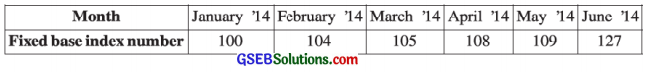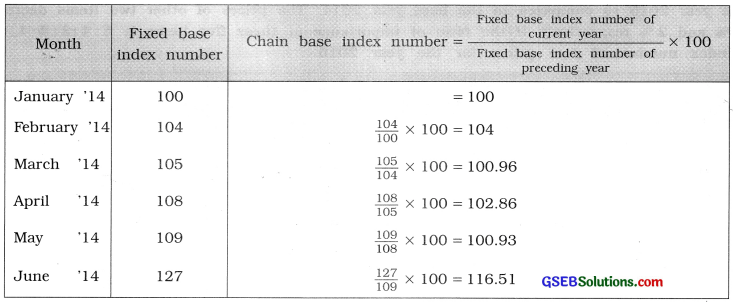Question 10.
Find the fixed base index numbers from the chain base index numbers given below: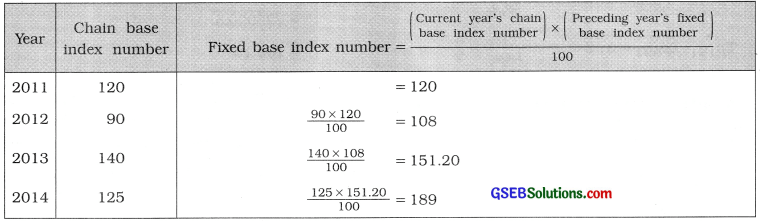Question 11.
Find the chain base index numbers from the following data regarding the price of an item: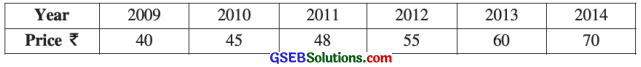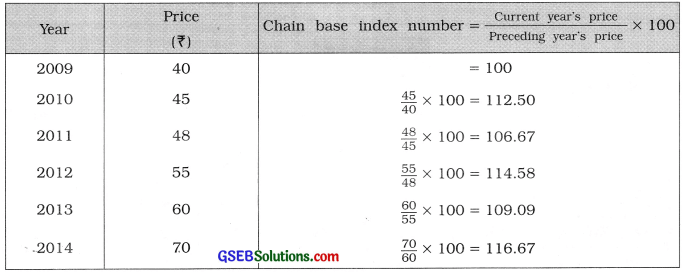Question 12.
Find the cost of living index number from the given information for the month of April, 2015 regarding group index numbers and weights of items of living of industrial workers.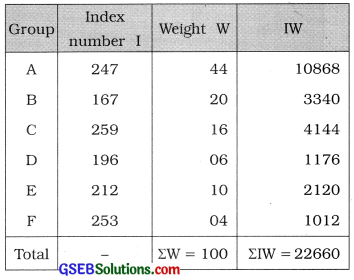Cost of living index number = $$\frac{\Sigma \mathrm{IW}}{\Sigma \mathrm{W}}$$
= $$\frac{22660}{100}$$
= 226.6

Question 13.
If Σp1 q0: Σp0 q0 = 5 : 3 and Σp1 q1: Σp0 q1 = 3:2, compute the Laspeyre’s, Paasche’s and Fisher’s index numbers.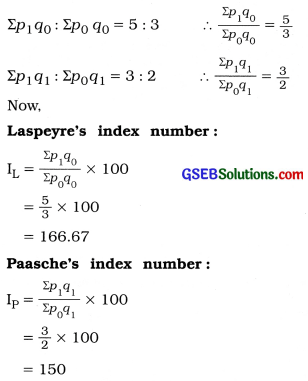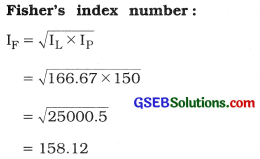Question 14.
If the ratio of Laspeyre’s and Paasche’s index number is 4:5 and Fisher’s index number is 150, find Paasche’s index number.
Here, IL : IP = 4 : 5;
IF = 150,
IP = ?
∴ $$\frac{I_{L}}{I_{P}}$$ = $$\frac{4}{5}$$
∴ IL = $$\frac{4}{5}$$IP
Now, IF = $$\sqrt{I_{L} \times I_{P}}$$
∴ 150 = $$\sqrt{\frac{4}{5} \mathrm{I}_{\mathrm{P}} \times \mathrm{I}_{\mathrm{P}}}$$
∴ (150)2 = $$\frac{4}{5}$$IP2
∴ $$\frac{22500 \times 5}{4}$$ = IP2
∴ IP2 = 28125
∴ IP2 = 167.71

Section E

Solve the following:

Question 1.
Find the general index number using the following data about prices of different items in the year 2012 by taking the base year 2010.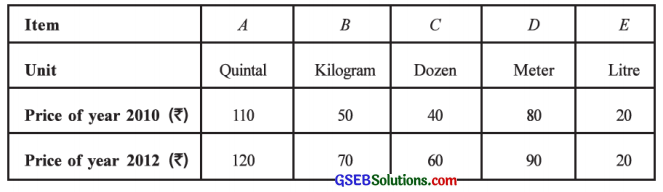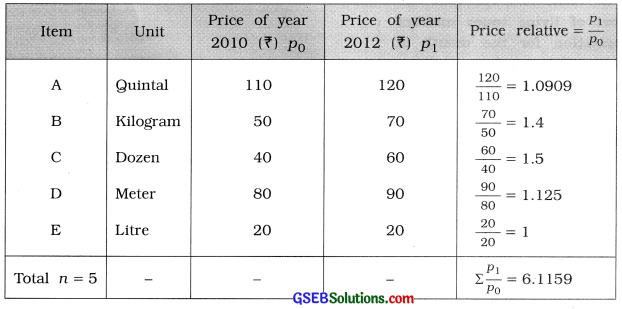General Index number = $$\frac{\sum\left(\frac{p_{1}}{p_{0}}\right)}{n}$$ × 100
= $$\frac{6.1159}{5}$$ × 100
= 122.32Question 2.
Compute the index number for the year 2015 with the base year 2010 by the method of total expenditure using the following data: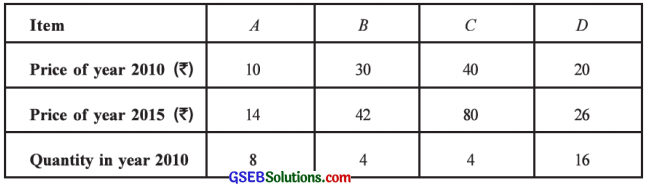Here, base year is 2010 and current year is 2015.
∴ p0 = Price in 2010; q0 = Quantity in 2010 and p1 = Price in 2015
The table for calculation is prepared as follows:Index number by total expenditure method = $$\frac{\Sigma p_{1} q_{0}}{\Sigma p_{0} q_{0}}$$ × 100 = $$\frac{1016}{680}$$ × 100 = 149.41

Question 3.
Compute the index number by the method of total expenditure for the year 2014 by taking the base year 2013 using the following data: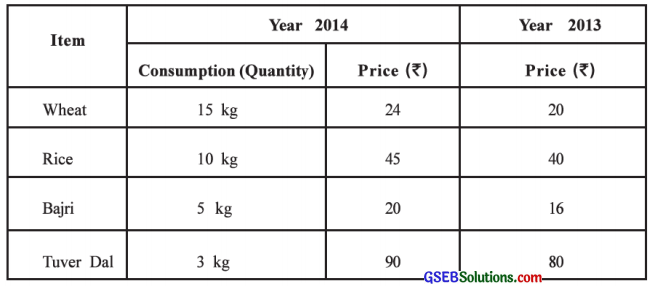Here, base year is 2013 and current year is 2014.
∴ p0 = Price in 2013; p1 = Price in 2014 and q1 = Quantity in 2014
The table for calculation is prepared as follows: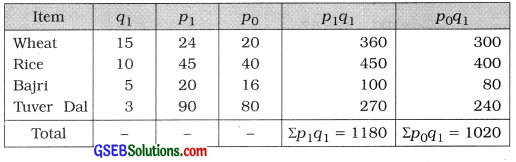Index number by total expenditure method = $$\frac{\Sigma p_{1} q_{1}}{\Sigma p_{0} q_{1}}$$ × 100
= $$\frac{1180}{1020}$$ × 100 = 115.69Question 4.
Use the following information to find (i) fixed base index numbers with the year 2008 as the base year and (ii) the index numbers by taking the average price of the years 2008 and 2009 as the base year price: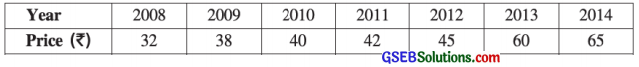(i) Base year = 2008(ii) Base year = Average of prices of the year 2008 and 2009:
Average price of 2008 and 2009 p0 = $$\frac{(\text { Price of } 2008)+(\text { Price of } 2009)}{2}$$
= $$\frac{32+38}{2}$$
= $$\frac{70}{2}$$ = ₹ 35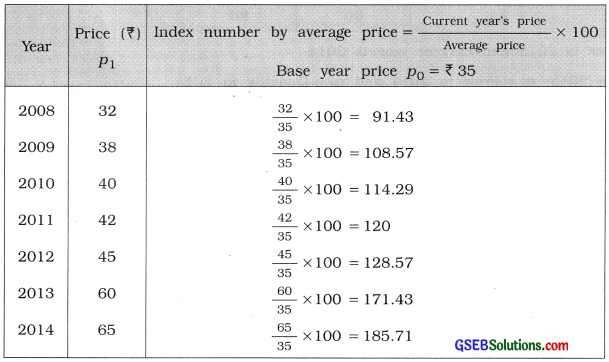Question 5.
The index numbers of different groups of industrial output of a city and the weights of these groups are given below. Find the index number of the industrial production.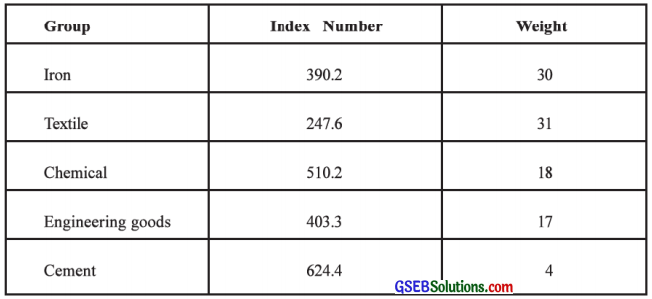Index number of industrial production I = $$\frac{\Sigma \mathrm{IW}}{\Sigma \mathrm{W}}$$
= $$\frac{37918.9}{100}$$
= 379.19

Question 6.
The price of wheat increased by 70 % and price of rice increased by 40 % in the year 2015 with respect to the year 2010. The price of bajri decreased by 25 %. The price of oil increased by 40 % and the price of ghee decreased by 5 %. If the importance of oil is three times and of rice is double that of ghee and the importance of each of wheat and bajri is double that of rice, find price index number of the group of these five items and interpret it.
Here, percentage increase or decrease in the prices of food items are given.
∴ Index number of the item = 100 + % increase OR
= 100 – % decrease
∴ Suppose, importance of ghee is 1.
∴ the importance of oil is 3 × 1 = 3 and that of rice is 2 × 1 = 2
The importance of wheat and bajri each is double the importance of rice,
∴ the importance of wheat is 2 × 2 = 4 and that of bajri is 2 × 2 = 4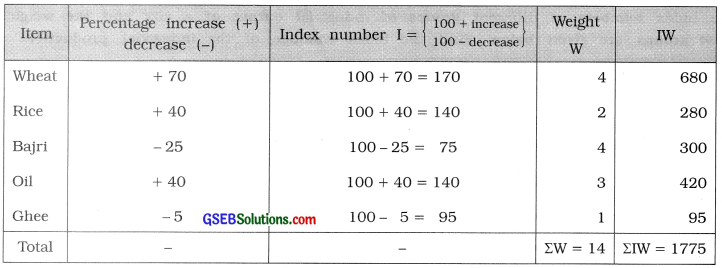General index number of price I = $$\frac{\Sigma I W}{\Sigma W}$$
= $$\frac{1775}{14}$$ = 126.79
Hence, price index of a group of five items is obtained 126.79.
Interpretation: There is 26.79 % in the prices of five items of the group.Question 7.
Calculate the real wages of a worker class from the following data about their monthly wages. Find the purchasing power of money in the year 2015 considering the year 2008 as the base year.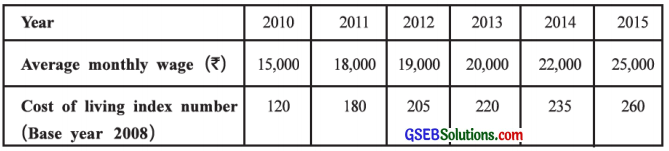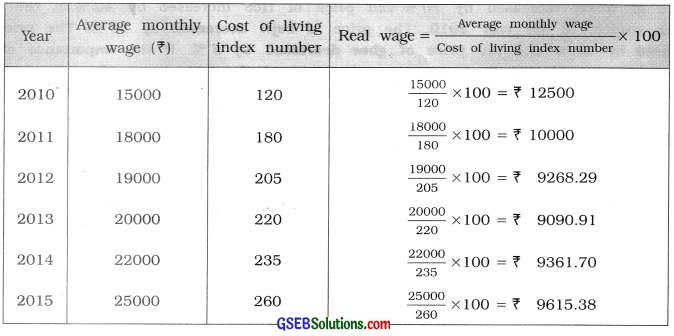Base year 2008, cost of living index number for the year 2015 is 260
∴ Purchasing power of money in the year 2015 = $$\frac{1}{\text { Cost of living index number }}$$ × 100
= $$\frac{1}{260}$$ × 100
= $$\frac{100}{260}$$ = ₹ 0.38

Section F

Solve the following:

Question 1.
Find the Laspeyre’s and Paasche’s index numbers using the following data for the year 2015 by taking the year 2014 as the base year. Also find the Fisher’s index number and interpret it.Here, price of items and its total expenditure are given. We obtain the consumption (quantity) of items using the formula, Consumption = $$\frac{\text { Total expenditure }}{\text { Price per unit }}$$

We take p0 = Price in 2014, p1 = Price in 2015, q0 = Quantity in 2014 and q1 = Quantity in 2015.
The table for calculation is prepared as follows:Laspeyre’s index number:
IL = $$\frac{\Sigma p_{1} q_{0}}{\Sigma p_{0} q_{0}}$$ × 100
= $$\frac{1132}{996}$$ × 100
= 113.65

Paasche’s index number:
Ip = $$\frac{\Sigma p_{1} q_{1}}{\Sigma p_{0} q_{1}}$$ × 100
= $$\frac{1430}{1255}$$ × 100
= 113.94

Fisher’s index number:
IF = $$\sqrt{\mathrm{I}_{\mathrm{L}} \times \mathrm{I}_{\mathrm{P}}}$$
= $$\sqrt{113.65 \times 113.94}$$
= $$\sqrt{12949.281}$$
= 113.79Question 2.
The quantity consumed and total expenditure of four different items are as given below. Find Paasche’s and Fisher’s index numbers for the year 2015 with respect to the year 2013.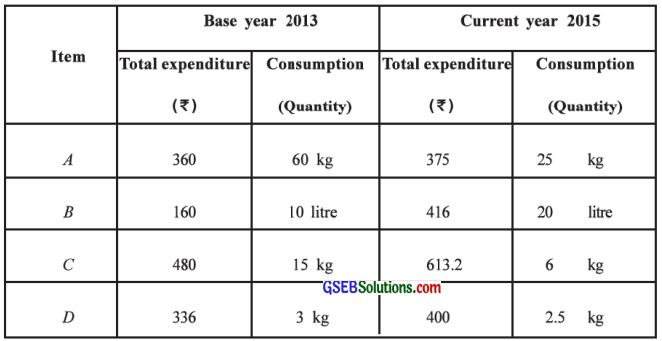Here, quantity (consumption) of items and their total expenditure are given. We obtain the price per unit of item using the formula, Price per unit = $$\frac{\text { Total expenditure }}{\text { Quantity }}$$

We take p0 = Price in 2013, p1 = Price in 2015, q0 = Quantity in 2013 and q1 = Quantity in 2015. The table for calculation is prepared as follows: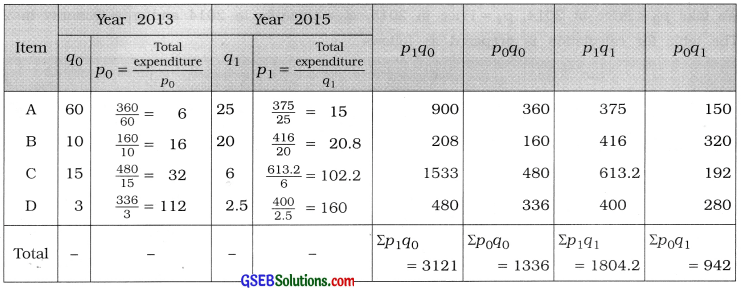Paasche’s index number:
Ip = $$\frac{\Sigma p_{1} q_{1}}{\Sigma p_{0} q_{1}}$$
= $$\frac{1804.2}{942}$$
= 1.53

Fisher’s index number:
IF = $$\sqrt{\frac{\Sigma p_{1} q_{0}}{\Sigma p_{0} q_{0}} \times \frac{\Sigma p_{1} q_{1}}{\Sigma p_{0} q_{1}}}$$ × 100
= $$\sqrt{\frac{3121}{1336} \times \frac{1804.2}{942}}$$ × 100
= $$\sqrt{\frac{5630908.2}{1258512}}$$ × 100
= $$\sqrt{4.4742}$$ × 100
= 2.1152 × 100
= 211.52

Question 3.
Compute the Fisher’s index number from the data given below about six different items:Here, base year is 2013 and current year is 2015.
∴ p0 – Price in 2013, q0 = Quantity in 2013, p1 = Price in 2015 and q1 = Quantity in 2015
The units of price and quantity for items A, B, C and F are not equal. We compute after making them equal.

Explanation: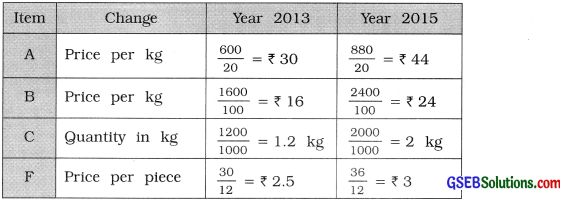The table for calculation is prepared as follows: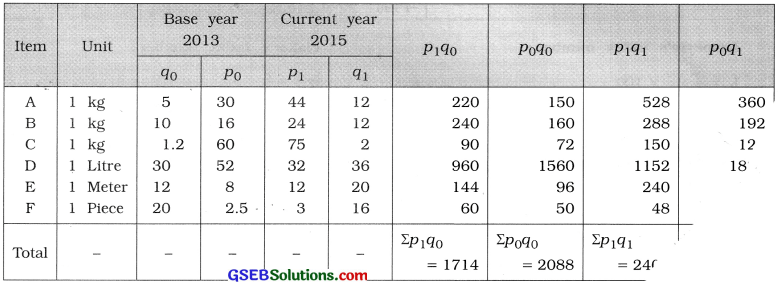Fisher’s index number:
IF = $$\sqrt{\frac{\Sigma p_{1} q_{0}}{\Sigma p_{0} q_{0}} \times \frac{\Sigma p_{1} q_{1}}{\Sigma p_{0} q_{1}}}$$ × 100
= $$\sqrt{\frac{1714}{2088} \times \frac{2406}{2744}}$$ × 100
= $$\sqrt{\frac{4123884}{5729472}}$$ × 100
= $$\sqrt{0.7198}$$ × 100
= 0.8484 × 100
= 84.84Question 4.
Compute the Laspeyre’s, Paasche’s and Fisher’s index numbers for the year 2015 from the data given below: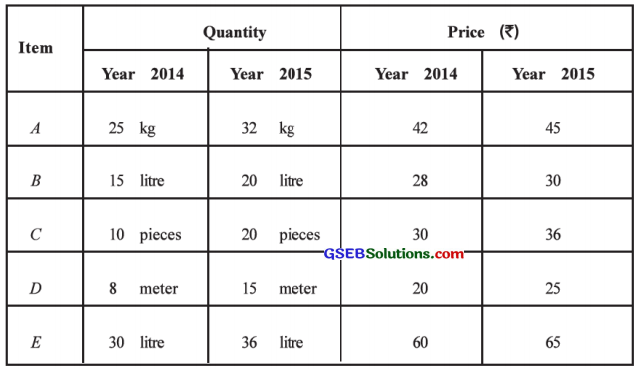Here, base year is 2014 and current year is 2015.
∴ P0 = Price in 2014, q0 = Quantity in 2014, P1 = Price in 2015 and q1 = Quantity in 2015
The table for calculation is prepared as follows: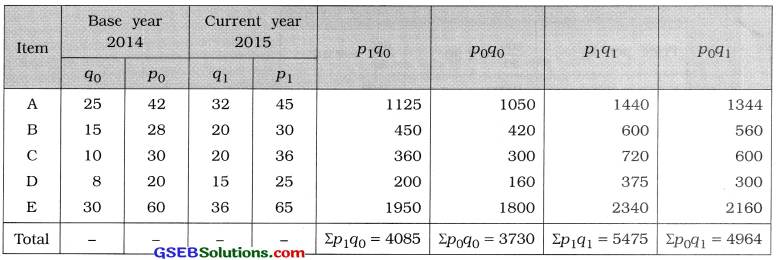Laspeyre’s index number:
IL = $$\frac{\Sigma p_{1} q_{0}}{\Sigma p_{0} q_{0}}$$ × 100
= $$\frac{4085}{3730}$$ × 100
= 109.52

Paasche’s index number:
= $$\frac{\Sigma p_{1} q_{1}}{\Sigma p_{0} q_{1}}$$ × 100
= $$\frac{5475}{4964}$$ × 100
= 110.29

Fisher’s index number:
IF = $$\sqrt{I_{L} \times I_{P}}$$
= $$\sqrt{109.52 \times 110.29}$$
= $$\sqrt{12078.96}$$
= 109.90

Question 5.
Compute the index number for the year 2015 by total expenditure method and family budget method and state whether both the index numbers are same: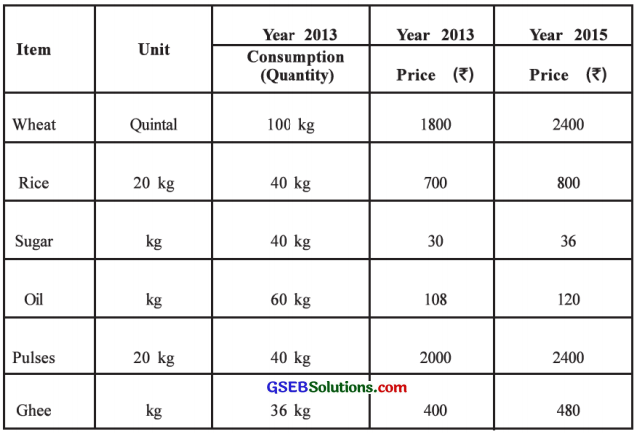Here, base year is 2013 and current year is 2015.
∴ p0 = Price in 2013, q0 = Quantity in 2013, p1 = Price in 2015 and q1 = Quantity in 2015 The units of price and quantity for the items wheat, rice and pulses are not equal. We compute the index number for the year 2015 by total expenditure method and family budget method after making the units of these items equal.

Explanation :The table for calculation is prepared as follows: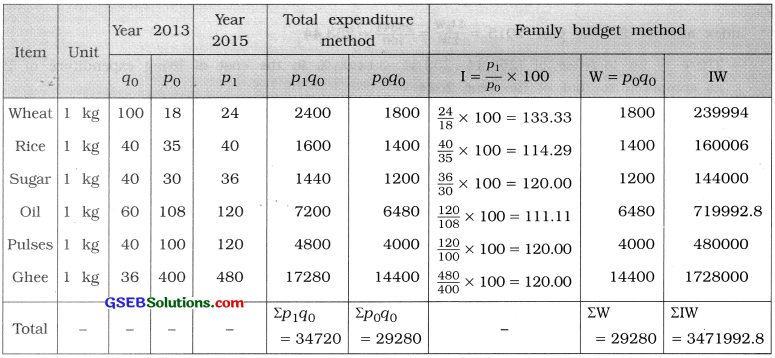Total expenditure method:
Index number for the year 2015
= $$\frac{\Sigma p_{1} q_{0}}{\Sigma p_{0} q_{0}}$$ × 100
= $$\frac{34720}{29280}$$ × 100
= 118.58

Family budget method:
Index number for the year 2015
= $$\frac{\Sigma \mathrm{IW}}{\Sigma \mathrm{W}}$$
= $$\frac{3471992.8}{29280}$$
= 118.58
Thus, the index numbers obtained by total expenditure method and family budget method are equal.Question 6.
The data about index numbers and weights for groups of items for the living of industrial workers in Ahmedabad city in the years 2014 and 2015 are as follows. Find the cost of living index number of industrial workers. If the wages of workers are increased by 5 % in the year 2015 then is this rise sufficient to compensate the rise in price in the year 2015 ?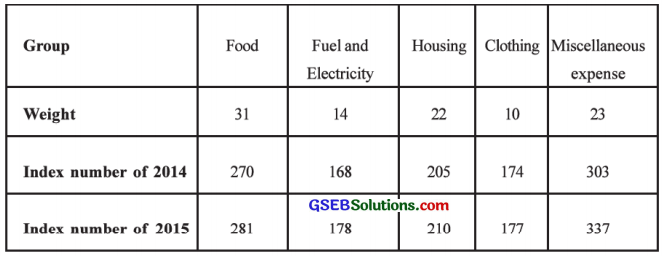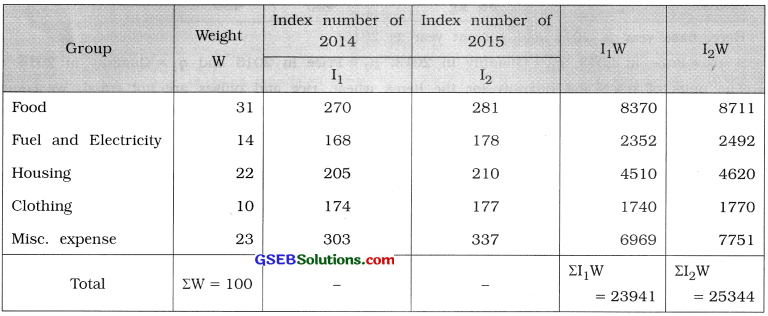Index number for the year 2014 = $$\frac{\Sigma \mathrm{I}_{1} \mathrm{~W}}{\Sigma \mathrm{W}}$$ = $$\frac{23941}{100}$$ = 239.41
Index number for the year 2015 = $$\frac{\Sigma \mathrm{2}_{1} \mathrm{~W}}{\Sigma \mathrm{W}}$$ = $$\frac{25344}{100}$$ = 253.44

→ There is an increase of (253.44 – 239.41 =) 14.03 % in the cost of living expenditure of 2015 as compared to that of the year 2014.

→ The wages of workers are increased by 5% in the year 2015.
The actual percentage increase in the cost of living expenditure of workers in the year 2015 as compared to the year 2014
= $$\frac{\text { Percentage increase in cost of living expenditure in the year } 2015}{\text { Cost of living index number for the year } 2014}$$ × 100
= $$\frac{14.03}{239.41}$$ × 100
= 0.0586 × 100
= 5.86 %
But the increase in wage is 5 %.
Hence, the increase in the wages of workers in the year 2015 is not sufficient and is short by (5.86 – 5 =) 0.86 %.

Question 7.
The following data are given about the index numbers and weights for the items of living of industrial workers in a city in the year 2014. Find the cost of living index number for industrial workers. If the average monthly salary paid to these workers in the year 2012 was ₹ 6,000, what should be the monthly salary in the current year 2014 to maintain the same standard of living?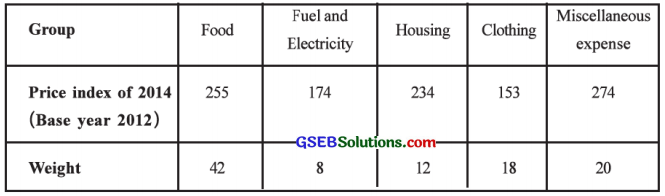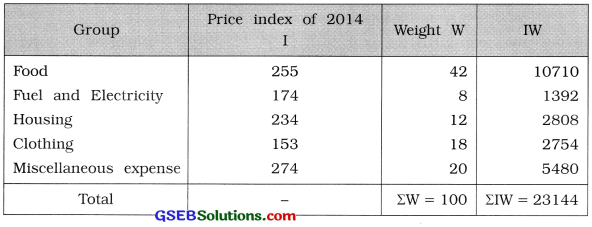Cost of living index number for industrial workers I = $$\frac{\Sigma \mathrm{IW}}{\Sigma \mathrm{W}}$$
= $$\frac{23144}{100}$$
= 231.44

→ Base year = 2012
There is (231.44 – 100 =) 131.44% increase in the cost of living expenditure in 2014 as compared to the base year 2012.

→ The average monthly salary of the workers in the year 2012 is ₹ 6,000.
∴ The average monthly salary in the current year 2014 to maintain same standard of living
= $$\frac{\text { Current year’s }(\text { i.e. } 2014) \text { cost of living index number }}{\text { Base year’s (i.e. 2012) cost of living index number }}$$ × Average salary in the year 2012
= $$\frac{231.44}{100}$$ × 6000
= ₹ 13886.40Question 8.
The data about the industrial production quantity and weights for the year 2015 are given below. Compute the index number of industrial production and interpret it.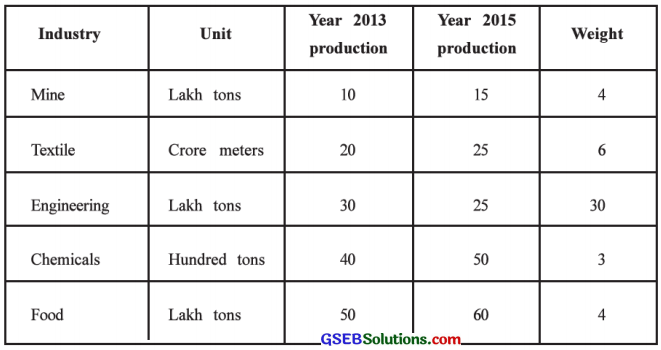Index number of industrial production I = $$\frac{\Sigma \mathrm{IW}}{\Sigma \mathrm{W}}$$
= $$\frac{4705}{47}$$
= 100.10
Interpretation: There is (100.10 – 100=) 0.10% increase in the industrial production of the year 2015 as compared to the year 2013.

Question 9.
The data about per unit price and weights of four different items in the year 2014 and 2015 are as follows. Compute the index number of the year 2015.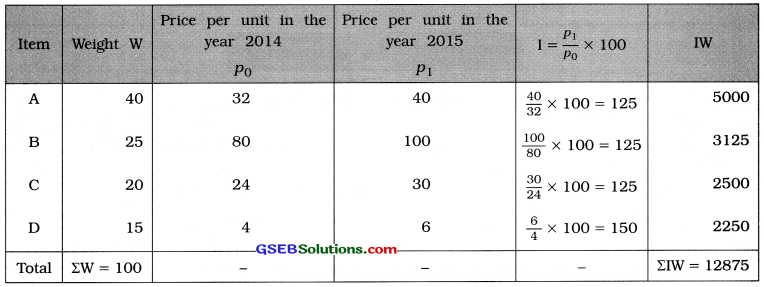Index number of the year 2015
I = $$\frac{\Sigma \mathrm{IW}}{\Sigma \mathrm{W}}$$
= = $$\frac{12875}{100}$$
= 128.75Question 10.
The index numbers of food and clothing among the different groups of cost of living are 150 and 224.7 respectively for the year 2015. The price of fuel has increased by 220%. The expense on rent has increased from ₹ 4000 to ₹ 6000 and miscellaneous expenses increased by 1.75 times. If the expenditure for the first four groups are 40%, 18%, 12 % and 20 % respectively, find the cost of living index number for the year 2015 and interpret it.
→ Index number of rent for the year 2015 = $$\frac{6000}{4000}$$ × 100 = 150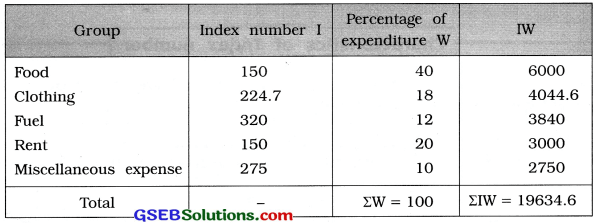Cost of living index number for the year 2015 I = $$\frac{\Sigma \mathrm{IW}}{\Sigma \mathrm{W}}$$
= $$\frac{19634.6}{100}$$